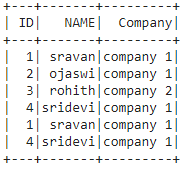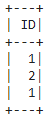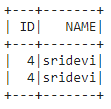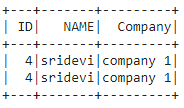# Select Columns that Satisfy a Condition in PySpark

• Last Updated : 29 Jun, 2021

In this article, we are going to select columns in the dataframe based on the condition using the where() function in Pyspark.

Let’s create a sample dataframe with employee data.

## Python3

 `# importing module``import` `pyspark`` ` `# importing sparksession from pyspark.sql module``from` `pyspark.sql ``import` `SparkSession`` ` `# creating sparksession and giving an app name``spark ``=` `SparkSession.builder.appName(``'sparkdf'``).getOrCreate()`` ` `# list  of employee data``data ``=` `[[``1``, ``"sravan"``, ``"company 1"``], [``2``, ``"ojaswi"``, ``"company 1"``],``        ``[``3``, ``"rohith"``, ``"company 2"``], [``4``, ``"sridevi"``, ``"company 1"``], ``        ``[``1``, ``"sravan"``, ``"company 1"``], [``4``, ``"sridevi"``, ``"company 1"``]]`` ` `# specify column names``columns ``=` `[``'ID'``, ``'NAME'``, ``'Company'``]`` ` `# creating a dataframe from the lists of data``dataframe ``=` `spark.createDataFrame(data, columns)`` ` `# display dataframe``dataframe.show()`

Output:### The where() method

This method is used to return the dataframe based on the given condition. It can take a condition and returns the dataframe

Syntax:

`where(dataframe.column condition)`
1. Here dataframe is the input dataframe
2. The column  is the column name where we have to raise a condition

### The select() method

After applying the where clause, we will select the data from the dataframe

Syntax:

`dataframe.select('column_name').where(dataframe.column condition)`
1. Here dataframe is the input dataframe
2. The column is the column name where we have to raise a condition

Example 1: Python program to return ID based on condition

## Python3

 `# importing module``import` `pyspark`` ` `# importing sparksession from pyspark.sql module``from` `pyspark.sql ``import` `SparkSession`` ` `# creating sparksession and giving an app name``spark ``=` `SparkSession.builder.appName(``'sparkdf'``).getOrCreate()`` ` `# list  of employee data``data ``=` `[[``1``, ``"sravan"``, ``"company 1"``], [``2``, ``"ojaswi"``, ``"company 1"``], ``        ``[``3``, ``"rohith"``, ``"company 2"``], [``4``, ``"sridevi"``, ``"company 1"``], ``        ``[``1``, ``"sravan"``, ``"company 1"``], [``4``, ``"sridevi"``, ``"company 1"``]]`` ` `# specify column names``columns ``=` `[``'ID'``, ``'NAME'``, ``'Company'``]`` ` `# creating a dataframe from the lists of data``dataframe ``=` `spark.createDataFrame(data, columns)`` ` `# select ID where ID less than 3``dataframe.select(``'ID'``).where(dataframe.``ID` `< ``3``).show()`

Output:Example 2: Python program to select ID and name  where ID =4.

## Python3

 `# importing module``import` `pyspark`` ` `# importing sparksession from pyspark.sql module``from` `pyspark.sql ``import` `SparkSession`` ` `# creating sparksession and giving an app name``spark ``=` `SparkSession.builder.appName(``'sparkdf'``).getOrCreate()`` ` `# list  of employee data``data ``=` `[[``1``, ``"sravan"``, ``"company 1"``], [``2``, ``"ojaswi"``, ``"company 1"``],``        ``[``3``, ``"rohith"``, ``"company 2"``], [``4``, ``"sridevi"``, ``"company 1"``], ``        ``[``1``, ``"sravan"``, ``"company 1"``], [``4``, ``"sridevi"``, ``"company 1"``]]`` ` `# specify column names``columns ``=` `[``'ID'``, ``'NAME'``, ``'Company'``]`` ` `# creating a dataframe from the lists of data``dataframe ``=` `spark.createDataFrame(data, columns)`` ` `# select ID and name  where ID =4``dataframe.select([``'ID'``, ``'NAME'``]).where(dataframe.``ID` `=``=` `4``).show()`

Output:Example 3: Python program to select all column based on condition

## Python3

 `# importing module``import` `pyspark`` ` `# importing sparksession from pyspark.sql module``from` `pyspark.sql ``import` `SparkSession`` ` `# creating sparksession and giving an app name``spark ``=` `SparkSession.builder.appName(``'sparkdf'``).getOrCreate()`` ` `# list  of employee data``data ``=` `[[``1``, ``"sravan"``, ``"company 1"``], [``2``, ``"ojaswi"``, ``"company 1"``],``        ``[``3``, ``"rohith"``, ``"company 2"``], [``4``, ``"sridevi"``, ``"company 1"``], ``        ``[``1``, ``"sravan"``, ``"company 1"``], [``4``, ``"sridevi"``, ``"company 1"``]]`` ` `# specify column names``columns ``=` `[``'ID'``, ``'NAME'``, ``'Company'``]`` ` `# creating a dataframe from the lists of data``dataframe ``=` `spark.createDataFrame(data, columns)`` ` `# select all columns e  where name = sridevi``dataframe.select([``'ID'``, ``'NAME'``, ``'Company'``]).where(``    ``dataframe.NAME ``=``=` `'sridevi'``).show()`

Output:My Personal Notes arrow_drop_up